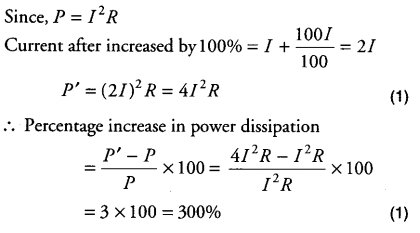# If the current I through a resistor is increased by 100% (assume that temperature

If the current I through a resistor is increased by 100% (assume that temperature remains unchanged), find the increase in power dissipated.Why cant we use the formula P=VI… The answer will be 100% then

Because if you increase current with a constant voltage then your resistance would reduce. Since there is a variable quantity we can’t use P=VI

Hello brother.
When you use VI you have to be careful about voltage also.
Resistance being constant.
V is directly proportional to I
So V will also be twice.
Now putting in formula
P=V’I’=2V2I=4VI.
Now you will get the same result i.e. 300%

Brother assume if you have a constant voltage V and you have to increase current I. Then, what would you do is to reduce resistance R. Then only would you be able to increase current.

And by the way, voltage is not directly proportional to current, CURRENT IS DIRECTLY PROPORTIONAL TO VOLTAGE.
If you change current, voltage WILL NOT change but if you change voltage, then, current WILL CHANGE.